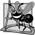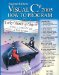Manipulations of class objects are accomplished by sending messages (in the form of method calls) to the objects. This method-call notation is cumbersome for certain kinds of classes, especially mathematical classes. For these classes, it would be convenient to use C#'s rich set of built-in operators to specify object manipulations. In this section, we show how to enable these operators to work with class objectsvia a process called operator overloading.## Software Engineering Observation 11 9

Use operator overloading when it makes an application clearer than accomplishing the same operations with explicit method calls.

C# enables you to overload most operators to make them sensitive to the context in which they are used. Some operators are overloaded frequently, especially the various arithmetic operators, such as + and -. The job performed by overloaded operators also can be performed by explicit method calls, but operator notation often is more natural. Figures 11.17 and 11.18 provide an example of using operator overloading with a ComplexNumber class.

Figure 11.17. Class that overloads operators for adding, subtracting and multiplying complex numbers.

 ``` 1 // Fig. 11.17: ComplexNumber.cs 2 // Class that overloads operators for adding, subtracting 3 // and multiplying complex numbers. 4 using System; 5 6 public class ComplexNumber 7 { 8 private double real; // real component of the complex number 9 private double imaginary; // imaginary component of the complex number 10 11 // constructor 12 public ComplexNumber( double a, double b ) 13 { 14 real = a; 15 imaginary = b; 16 } // end constructor 17 18 // return string representation of ComplexNumber 19 public override string ToString() 20 { 21 return string.Format( "({0} {1} {2}i)", 22 Real, ( Imaginary < 0 ? "-" : "+" ), Math.Abs( Imaginary ) ); 23 } // end method ToString 24 25 // read-only property that gets the real component 26 public double Real 27 { 28 get 29 { 30 return real; 31 } // end get 32 } // end property Real 33 ``` 34 // read-only property that gets the imaginary component 35 public double Imaginary 36 { 37 get 38 { 39 return imaginary; 40 } // end get 41 } // end property Imaginary 42 43 // overload the addition operator 44 public static ComplexNumber operator+( 45 ComplexNumber x, ComplexNumber y ) 46 { 47 return new ComplexNumber( x.Real + y.Real, 48 x.Imaginary + y.Imaginary ); 49 } // end operator + 50 51 // overload the subtraction operator 52 public static ComplexNumber operator-( 53 ComplexNumber x, ComplexNumber y ) 54 { 55 return new ComplexNumber( x.Real - y.Real, 56 x.Imaginary - y.Imaginary ); 57 } // end operator - 58 59 // overload the multiplication operator 60 public static ComplexNumber operator*( 61 ComplexNumber x, ComplexNumber y ) 62 { 63 return new ComplexNumber( 64 x.Real * y.Real - x.Imaginary * y.Imaginary, 65 x.Real * y.Imaginary + y.Real * x.Imaginary ); 66 } // end operator * 67 } // end class ComplexNumber

(This item is displayed on page 547 in the print version)

``` 1 // Fig 11.18: OperatorOverloading.cs
3 using System;
4
5 public class ComplexTest
6 {
7 public static void Main( string[] args )
8 {
9 // declare two variables to store complex numbers
10 // to be entered by user
11 ComplexNumber x, y;
12
13 // prompt the user to enter the first complex number
14 Console.Write( "Enter the real part of complex number x: " );
15 double realPart = Convert.ToDouble( Console.ReadLine() );
16 Console.Write(
17 "Enter the imaginary part of complex number x: " );
18 double imaginaryPart = Convert.ToDouble( Console.ReadLine() );
19 x = new ComplexNumber( realPart, imaginaryPart );
20
21 // prompt the user to enter the second complex number
22 Console.Write( "
Enter the real part of complex number y: " );
23 realPart = Convert.ToDouble( Console.ReadLine() );
24 Console.Write(
25 "Enter the imaginary part of complex number y: " );
26 imaginaryPart = Convert.ToDouble( Console.ReadLine() );
27 y = new ComplexNumber( realPart, imaginaryPart );
28
29 // display the results of calculations with x and y
30 Console.WriteLine();
31 Console.WriteLine( "{0} + {1} = {2}", x, y, x + y );
32 Console.WriteLine( "{0} - {1} = {2}", x, y, x - y );
33 Console.WriteLine( "{0} * {1} = {2}", x, y, x * y );
34 } // end method Main
35 } // end class ComplexTest
```

 ```Enter the real part of complex number x: 2 Enter the imaginary part of complex number x: 4 Enter the real part of complex number y: 4 Enter the imaginary part of complex number y: -2 (2 + 4i) + (4 - 2i) = (6 + 2i) (2 + 4i) - (4 - 2i) = (-2 + 6i) (2 + 4i) * (4 - 2i) = (16 + 12i) ```

Class ComplexNumber (Fig. 11.17) overloads the plus (+), minus (-) and multiplication (*) operators to enable programs to add, subtract and multiply instances of class ComplexNumber using common mathematical notation. Lines 89 declare instance variables for the real and imaginary parts of the complex number.

Lines 4449 overload the plus operator (+) to perform addition of ComplexNumbers. Keyword operator, followed by an operator symbol, indicates that a method overloads the specified operator. Methods that overload binary operators must take two arguments. The first argument is the left operand, and the second argument is the right operand. Class ComplexNumber's overloaded plus operator takes two ComplexNumber references as arguments and returns a ComplexNumber that represents the sum of the arguments. Note that this method is marked public and static, which is required for overloaded operators. The body of the method (lines 4748) performs the addition and returns the result as a new ComplexNumber. Notice that we do not modify the contents of either of the original operands passed as arguments x and y. This matches our intuitive sense of how this operator should behaveadding two numbers does not modify either of the original numbers. Lines 5266 provide similar overloaded operators for subtracting and multiplying ComplexNumbers.## Software Engineering Observation 11 10

Overload operators to perform the same function or similar functions on class objects as the operators perform on objects of simple types. Avoid non-intuitive use of operators.## Software Engineering Observation 11 11

At least one argument of an overloaded operator method must be a reference to an object of the class in which the operator is overloaded. This prevents programmers from changing how operators work on simple types.

Class ComplexTest (Fig. 11.18) demonstrates the overloaded operators for adding, subtracting and multiplying ComplexNumbers. Lines 1427 prompt the user to enter two complex numbers, then use this input to create two ComplexNumbers and assign them to variables x and y.

Lines 3133 add, subtract and multiply x and y with the overloaded operators, then output the results. In line 31, we perform the addition by using the plus operator with ComplexNumber operands x and y. Without operator overloading, the expression x + y would not make sensethe compiler would not know how two objects should be added. This expression makes sense here because we've defined the plus operator for two ComplexNumbers in lines 4449 of Fig. 11.17. When the two ComplexNumbers are "added" in line 31 of Fig. 11.18, this invokes the operator+ declaration, passing the left operand as the first argument and the right operand as the second argument. When we use the subtraction and multiplication operators in lines 3233, their respective overloaded operator declarations are invoked similarly.

Notice that the result of each calculation is a reference to a new ComplexNumber object. When this new object is passed to the Console class's WriteLine method, its ToString method (lines 1923 of Fig. 11.17) is implicitly invoked. We do not need to assign an object to a reference-type variable to invoke its ToString method. Line 31 of Fig. 11.18 could be rewritten to explicitly invoke the ToString method of the object created by the overloaded plus operator, as in:

```Console.WriteLine( "{0} + {1} = {2}", x, y, ( x + y ).ToString() );
```Visual C# 2005 How to Program (2nd Edition)
ISBN: 0131525239
EAN: 2147483647
Year: 2004
Pages: 600